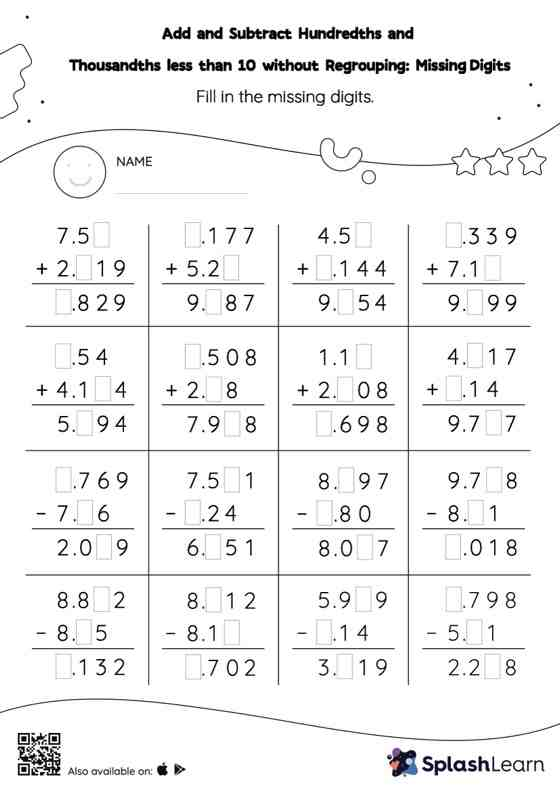# Add and Subtract Hundredths and Thousandths less than 10 without Regrouping: Missing Digits Worksheet

Home > Add and Subtract Hundredths and Thousandths less than 10 without Regrouping: Missing DigitsThis add and subtract hundredths and thousandths less than 10 without regrouping worksheet has a set of problems curated to ensure that your child becomes more proficient in addition and subtraction. Students find the missing number while adding and subtracting decimals by using the relationship between addition and subtraction. Add and subtract hundredths and thousandths less than 10 without regrouping worksheet does not require them to regroup numbers.